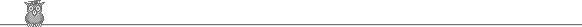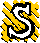### 36th Putnam 1975A1.  A triangular number is a positive integer of the form n(n + 1)/2. Show that m is a sum of two triangular numbers iff 4m + 1 is a sum of two squares.A2.  For what region of the real (a, b) plane, do both (possibly complex) roots of the polynomial z2 + az + b = 0 satisfy |z| < 1?A3.  Let 0 < α < β < γ ∈ R, the reals. Let K = { (x, y, z) : xβ + yβ + zβ = 1, and x, y, z ≥ 0} ∈ R3. Define f : K → R by f(x, y, z) = xα + yβ + zγ. At what points of K does f assume its maximum and minimum values?A4.  m > 1 is odd. Let n = 2m and θ = e2πi/n. Find a finite set of integers {ai} such that ∑ ai θi = 1/(1 - θ).A5.  Let I be an interval and f(x) a continuous real-valued function on I. Let y1 and y2 be linearly independent solutions of y'' = f(x) y, which take positive values on I. Show that from some positive constant k, k √(y1 y2) is a solution of y'' + 1/y3 = f(x) y.A6.  Given three points in space forming an acute-angled triangle, show that we can find two further points such that no three of the five points are collinear and the line through any two is normal to the plane through the other three.B1.  Let G be the group { (m, n) : m, n are integers } with the operation (a, b) + (c, d) = (a + c, b + d). Let H be the smallest subgroup containing (3, 8), (4, -1) and (5, 4). Let Hab be the smallest subgroup containing (0, a) and (1, b). Find a > 0 such that Hab = H.B2.  A slab is the set of points strictly between two parallel planes. Prove that a countable sequence of slabs, the sum of whose thicknesses converges, cannot fill space.B3.  Let n be a fixed positive integer. Let S be any finite collection of at least n positive reals (not necessarily all distinct). Let f(S) = (∑a∈S a)n, and let g(S) = the sum of all n-fold products of the elements of S (in other words, the nth symmetric function). Find supS g(S)/f(S).B4.  Does a circle have a subset which is topologically closed and which contains just one of each pair of diametrically opposite points?B5.  Define f0(x) = ex, fn+1(x) = x fn'(x). Show that ∑0∞ fn(1)/n! = ee.B6.  Let hn = ∑1n 1/r. Show that n - (n - 1) n-1/(n-1) > hn > n(n + 1)1/n - n for n > 2.To avoid possible copyright problems, I have changed the wording, but not the substance, of all the problems. The original text and official solutions were published in American Mathematical Monthly 83 (1976) 703-8. They are also available (with the solutions expanded) in: Gerald L Alexanderson et al, The William Lowell Putnam Mathematical Competition, 1965-1984. Out of print, but in some university libraries.

Putnam home